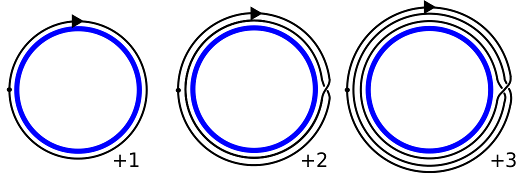### Highlights#### A chromatic look at the homotopy groups of spheres

MSRI - September 2015

A hallmark of algebraic topology is its collection of decades-old computational problems that have attracted considerable attention and yet remain unsolved. The most famous is the computation of the homotopy groups of spheres , which asks for a classification of all continuous functions S k S n from the k -dimensional sphere to the n -dimensional sphere up to continuous definition. When k < n , it turns out that any function S k S n can be deformed to a constant function, but when k n , this classification is highly non-trivial.

For example, a distinct equivalence class of functions S 1 S 1 can be defined for each integer m ∈ ℤ — geometrically the function takes the input circle and winds it m times around the target circle in a clockwise direction if m is positive and in a counterclockwise direction if m is negative — and there are no other continuous functions from the circle to itself.“Fundamental group of the circle.svg” by Salix, alba is used under the Creative Commons Attribution-ShareAlike 3.0 Unported License

The continuous functions S n S n can be characterized in the same manner, when the meaning of “winding” is generalized to higher dimensions.

A general classification of the continuous maps S n+k S n when k > 0 is not known and is the major outstanding problem in the field of algebraic topology. A theorem of Freudenthal implies that the group π S k of maps S n+k → S n is independent of n for n > k + 1. Making the computation of the stable homotopy groups of spheres seem particularly tractible is a result of Serre, which states that each unknown stable homotopy group is finite. The first few stable homotopy groups, displayed in the table below, follow no obvious pattern, and explicit values remain unknown for large k .

 π S 0 π S 1 π S 2 π S 3 π S 4 π S 5 π S 6 π S 7 π S 8 π S 9 π S 10 π S 11 ℤ ℤ/2 ℤ/2 ℤ/24 0 0 ℤ/2 ℤ/240 (ℤ/2) 2 (ℤ/2) 3 ℤ/6 ℤ/504
 π S 12 π S 13 π S 14 π S 15 π S 16 π S 17 π S 18 π S 19 0 ℤ/3 (ℤ/2) 2 ℤ/480 ⊕ ℤ/2 (ℤ/2) 2 (ℤ/2) 4 ℤ/8 ⊕ ℤ/2 ℤ/264 ⊕ ℤ/2

Because the unknown stable homotopy groups are finite, it is useful to look for patterns in the p-components , those maps that are annihilated by a prime p . Patterns occuring “one prime at a time” become more visible after localizing , an idea borrowed from number theory. The integers form a subset of the collection of fractions whose denominators are prime to p , which sit inside the collection of all rational numbers; that is, for every prime p there is a chain of localizations ℤ → ℤ (p) → ℚ. For the sphere spectrum S , the collection of spheres in all dimensions considered together, there exist similar localizations S → S (p) → Sℚ with one key difference: there is now an infinite tower of intermediate localizations

S → S (p) → ⋅⋅⋅ → SE(2) → SE(1) → Sℚ

called the chromatic tower . In homotopy theory, there is an “extra dimension of primes” which govern the intermediate layers between S (p) and S ℚ. One aim in chromatic homotopy theory is to study patterns in the stable homotopy groups of spheres that occur in “periodic families,” arising in recognizable patterns. Two of the major outstanding conjectures in stable homotopy theory, called the telescope and chromatic splitting conjectures , aim to describe the intermediate localizations and the so-called monochromatic layers , which explain how they fit together.

 References [MRW] Haynes R. Miller, Douglas C. Ravenel, and W. Stephen Wilson, Periodic phenomena in the Adams- Novikov spectral sequence, Ann. of Math. (2) 106 (1977), no. 3, 469 516. Date: June 3, 2015. [R86] Douglas C. Ravenel, Complex cobordism and stable homotopy groups of spheres, Pure and Applied Mathematics, vol. 121, Academic Press Inc., Orlando, FL, 1986. [R92] Douglas C. Ravenel, Nilpotence and periodicity in stable homotopy theory, Annals of Mathematics Studies, vol. 128, Princeton University Press, Princeton, NJ, 1992, Appendix C by Je Smith.